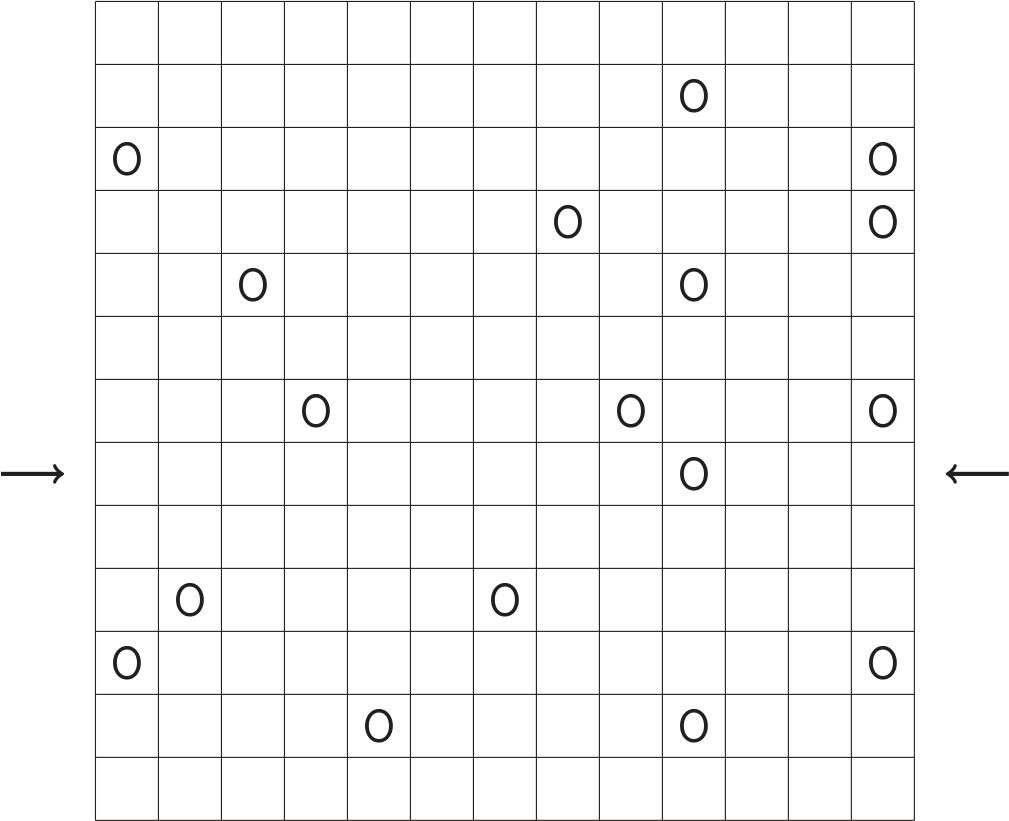# Prize crossnumber, Issue 15

Win a £100 Maths Gear goody bag by solving our infamous puzzleOur original prize crossnumber is featured on pages 60 and 61 of Issue 15.

### Rules

• Each of the letters $r$, $s$, $t$, $u$, $v$, $w$, $x$, $y$, and $z$ represents a different integer between 1 and 9 (inclusive).
• The clues are provided as normal, but the locations of black squares in the grid are not given. Instead, you are given the position of every zero in the completed crossnumber.
• The completed grid has order 4 rotational symmetry.
• The starting squares for the clues are numbered from 1 to 60 in the usual order: starting in the top left corner and proceeding left to right for each row.
• Solvers may wish to use the OEIS, Wikipedia, Python, an abacus, etc to (for example) obtain a list of cube numbers, but no programming should be necessary to solve the puzzle. As usual, no numbers begin with 0.
• One randomly selected correct answer will win a £100 Maths Gear goody bag, including non-transitive dice, a Festival of the Spoken Nerd DVD, and much, much more. Three randomly selected runners up will win a Chalkdust T-shirt. Maths Gear is a website that sells nerdy things worldwide, with free UK shipping.
• To enter, submit the sum of all the digits in the row marked by arrows using this form by 30 September 2022. Only one entry per person will be accepted. Winners will be notified by email and announced on our blog by 10 November 2022.

### Crossnumber

Crossnumber #15, set by Humbug:### Across clues

• 1. ${r}^3$ (3)
• 3. $(10+{r})^2$ (3)
• 5. $(8+{r})\times(20+{r})$ (3)
• 7. ${r}^2$ (2)
• 8. $11^{t}$ (3)
• 10. ${z}\times{r}\times{s}\times{t}$ (3)
• 12. ${z}^2$ (2)
• 14. $(60+{u})\times(190+{t})$ (5)
• 16. $2^{z}\times{r}-{s}$ (3)
• 18. ${t}\times{v}\times{w}\times{s}\times{z}$ (3)
• 20. $2520\div{r}$ (3)
• 21. $110\times{v}$ (3)
• 23. $337\times{x}$ (4)
• 25. $337\times{w}$ (4)
• 26. $792\div{y}$ (2)
• 27. $({r}+{x})^2$ (3)
• 29. $13\times{s}$ (3)
• 31. $2022\times{v}$ (4)
• 32. $677\times{w}$ (4)
• 34. $111\times{z}$ (3)
• 36. $({u}+{z})^2$ (3)
• 38. ${w}^3$ (2)
• 39. $1111\times{t}$ (4)
• 41. $67\times{r}\times{z}$ (4)
• 43. $606\div{x}$ (3)
• 44. $2520\div{w}$ (3)
• 45. $151\times{w}$ (3)
• 47. $(25-{w})\times(25+{w})$ (3)
• 49. $(110+{y})\times(110+{w})$ (5)
• 52. ${x}+{y}$ (2)
• 54. $14^{t}+{z}$ (3)
• 56. ${r}^3\times{t}$ (3)
• 57. ${u}^{t}$ (2)
• 58. ${t}^0+{t}^1+{t}^3+{t}^4+{t}^5+{t}^8+{t}^9$ (3)
• 59. $990\div{r}$ (3)
• 60. $(20+{y})\times(20-{y})$ (3)

### Down clues

• 1. ${r}\times3$ (2)
• 2. $2^{s}$ (3)
• 3. ${t}\times(100+{s})$ (3)
• 4. ${y}\times{z}$ (2)
• 5. $2424\div{y}$ (3)
• 6. ${s}\times{x}$ (2)
• 7. $29\times{z}$ (3)
• 9. $1111\times{t}$ (4)
• 11. $(10+{v})\times(330+{v})$ (4)
• 13. $100\times{s}$ (3)
• 14. ${u}\times{s}\times{r}$ (3)
• 15. $133\times{y}^2$ (4)
• 17. $11111\times{v}$ (5)
• 19. $3^{x}$ (3)
• 22. $110+{v}$ (3)
• 24. $2520\div{s}$ (3)
• 26. $103\times{s}$ (3)
• 27. $({w}+{y})^2$ (3)
• 28. $({t}+{s})^2$ (3)
• 30. $101\times{z}$ (3)
• 31. ${s}^{t}\times{z}\times{x}\times{y}$ (5)
• 33. $101\times{w}$ (3)
• 35. ${x}!$ (3)
• 37. ${z}^4$ (4)
• 38. ${u}^{u}-1$ (3)
• 40. $(50+{t})\times(50+{r})$ (4)
• 41. ${x}\times{w}\times{s}\times{z}\times{r}\div{t}$ (4)
• 42. $111\times{u}$ (3)
• 43. $99+{t}$ (3)
• 46. $102\times{w}$ (3)
• 48. $2^{z}-1$ (3)
• 50. $2^{x}\times{r}+{y}$ (3)
• 51. $(99+{t})\times({y}-{r})$ (3)
• 53. ${x}\times{y}$ (2)
• 55. $2^{r}-1$ (2)
• 57. ${t}^{u}$ (2)